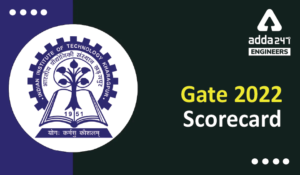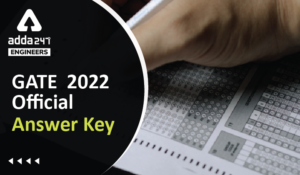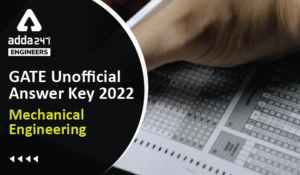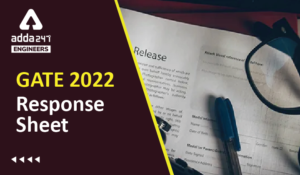Engineering Jobs   »   Electrical Engineering quizs   »   GATE 2022, ELECTRICAL GATE QUIZ,

# GATE’22 EE: Daily Practices Quiz 08-Sep-2021

GATE 2022 Important Dates: Organizing Institute for GATE 2022 is the Indian Institute of Technology Kharagpur. For GATE 2022 Exam IIT Kharagpur has released the official notification and Important dates regarding the GATE 2022 Exam. Aspirants preparing for GATE 2022 must check the details regarding GATE 2022 Important Dates. Read the complete article below for the information related to GATE 2022 Important dates.

GATE’22 EE: Daily Practices Quiz 08-Sep-2021

Each question carries 2 marks
Negative marking: 2/3 mark

Total Questions: 05

Total marks: 10
Time: 12 min.

Q1. An open loop control system results in a response of e^(-2t) (sin5t + cos5t) for a unit impulse input. The DC gain of the control system is ______.
(a) 241
(b) 0.241
(c)41
(d) 0.21

Q2. For a two-port network the three parameters A, B and C are given by—
A = x1,B = x2,C =1/x2
then D is equal to under the network to be reciprocal—
(a) x1
(b) 0
(c) 2/x(1 )
(d) 2×1

Q3. The incandescent bulbs respectively as P_1 and P_2 for operation at a specified main voltage are connected in series across the mains as shown in the figure, then the total power supplied by the mains to the two bulbs—(a) P1 P2
(b) P1^2+P2^2
(c) (P1 P2)/(P1+P2 )
(d) P1+P2

Q4. The time constant of the network shown in the fig. is—(a) 4RC
(b) 3RC
(c) 2RC
(d) 2RC/3

Q5. Calculate the Voltage regulation of the transformer in which ohmic loss is 1 % of the output and reactance drop is 5 % of the voltage when the power factor is 0.8 lag
(a) 1 %
(b) 3.5 %
(c) 3.8 %
(d) 5 %

SOLUTIONS

S1. Ans.(b)
Sol. g(t)=e^– 2t (sin5t + cos5t)
Taking Laplace transform; G(s)=5/((s+2)^2+5^2 )+(s+2)/((s+2)^2+5^2 )
For DC gain; |G(s)|_(s=0)
∴G(0)=5/((0+2)^2+5^2 )+(0+2)/((0+2)^2+5^2 )=5/29+2/29=7/29=0.241

S2. Ans.(c)
Sol. The condition of reciprocity for ABCD parameters is given by relation
∴x1 D-x2×1/x2 =1
∴x1 D=1+1=2
⇒D=2/x1

S3. Ans.(d)
Sol. Since bulbs are connected in series.
∴PT=I^2 (R1+R2 )=P1+P2

S4. Ans.(a)
Sol.Time constant τ = R_eq C
∵R_eq = 4R | | 4R = 2R
C = 2C
so, τ = 2 R. 2C
= 4RC

S5. Ans.(c)
Sol. here, %R=1 %
%X=5 %
Pf=cos?=0.8 lagging
∴VR=%R cosϕ+%X sinϕ=1*0.8+5*0.6=3.8 %(+ sign because of lagging pf)

Sharing is caring!

Thank You, Your details have been submitted we will get back to you.
•HURL Recruitment 2022 Apply Online for 1...
•GATE 2022 Scorecard Released, Check Step...
•GATE Result 2022 OUT Live Check Your GAT...
•GATE 2022 Official Answer Key Out, Downl...
•GATE 2022 Answer Key Mechanical Engineer...
•GATE 2022 Response Sheet, Direct Link to...